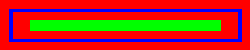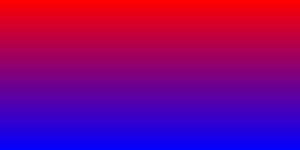# GD Rectangles

 This tutorial concentrates on rectangles. As in previous tutorials, the codes are similar for setting the background, colors, and line thickness. Rectangles can be drawn as either an outline or a filled rectangle. It is similar to lines in that we have to designate an x,y coordinate for the top left corner, and an x,y coordinate for the bottom right corner. This is used also to make squares, just configure it for a square. For an outline use: imagerectangle(\$image, x1,y1,x2,y2,\$color); For a filled rectangle use:imagefilledrectangle(\$image, x1,y1,x2,y2,\$color);The filled rectangle ignores the line thickness. Right click, select all, ctrl+c to copy. Right click, select all, ctrl+c to copy. \$x=-20; \$y=\$size+20; \$count=0; while(\$count<=5){ \$count=\$count+1; \$x=\$x+20; \$y=\$y-20; \$color = ImageColorAllocate(\$image, rand(0,255), rand(0,255), rand(0,255)); imagesetthickness(\$image,10); imagefilledrectangle(\$image, \$x,\$x,\$y,\$y,\$color); } The above code is using a "while loop" (as opposed to a for loop used previously). The while loop is similar to the for loop. I find it easier to work with. You'll notice that first the width and height are set to a variable called \$size. This allows me to use the variable in the loop. This loop is set to do something 5 times. The \$count is set to begin at 0 outside the loop. Inside the while() it is set to do something 5 times. Inside the loop {} I want the count to add 1 each time until it comes to 5, and then stop. I want to draw 5 rectangles. The first is the size of the background, and for each rectangle make it go in and down 20 pixels. So I need to add 20 to the x variables on the top left and subtract 20 pixels from the y variable on the lower right. It is set to a negative number because once the loop begins, it starts to add and subtract, so the first rectangle is drawn at 20,20,250,250, the second is drawn at 40,40,230,230, etc. The numbers that remain constant are written outside the loop, while the numbers that change are written inside the loop. The colors here are inside the loop because they are set to change each time the rectangle is drawn. rand(0,255) tells the server to pick a random number from zero to 255 for the red, green, and blue colors. rand is a php global type built-in variable. There is a header refresh on the first line which reloads the page. If you want to use it in a web page you need to embed the php URL. If I haven't mentioned it already, you can show any PHP GD image using an image tag such as Here is another example of rectangles with loops. I found this code in the PHP Manual on the web. You'll notice that the script uses a "nested for loop" which is a loop inside a loop, or 2 loops working at the same time. They both close at the same time with a curly bracket for each loop. Rectangle loopCode: rectangle textHere is another gradient script that uses rectangles instead of lines. It also has functions to convert Hex colors to RGB colors. There are notes on the page. Also the addy for the original page is on the top line. Just thought you might want it for future reference. I'm still trying to grasp the color convert thing. Rectangle Gradient text Some helpful color lookups. This one from Sally's site. RGB color A Dec to Hex converter for each number. Dec/Hex FYI: You can use hex colors inside the RGB command if you use a 0x before the number. In other words imagecolorallocate(\$image, 0xff,0xff,0xff); is the same as imagecolorallocate(\$image, 255,255,255);